Covalent, Carbon biochem botany 2020
Email *
Last Name *
First Name *
Period *
1. What is the maximum number of covalent bonds than an atom of oxygen can form?
2 points
2. Which hydrocarbon below would contain (1) one double bond and still be built correctly?
2 points
3. Refer to diagram 2 below. Which of the following molecules ARE built correctly?
2 points
diagram 2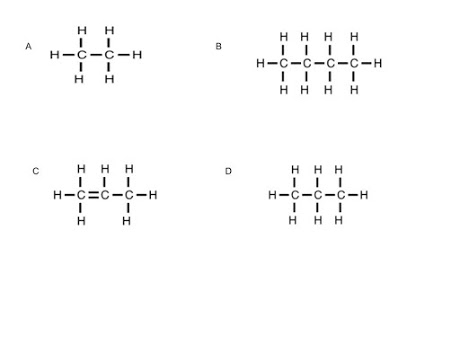4. Which molecules would be considered isomers, below in figure 3?
2 points
figure 3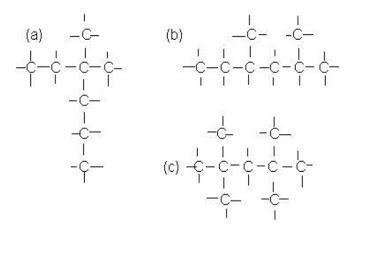4. Above, in figure 3, what is the correct molecular formula for the molecule in choice B?
2 points
5. C4H6 could be the the chemical formula for: check all that apply
2 points
9. 9.Use the electron dot diagrams below (diagram 4): If two atoms of X and Z were to covalently bond to each other, what molecule would result?
2 points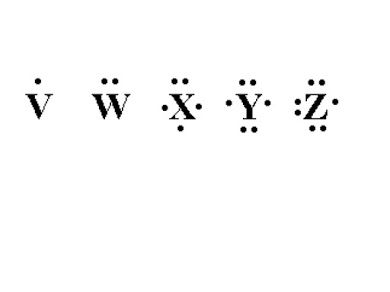10. If two atoms of Y could covalently bond together, what type of bond would result? Use diagram 4 above
2 points
11. Based on your knowledge of how carbon and hydrogen form covalent bonds, which of the hydrocarbon molecules below are built correctly? see diagram 4 below
2 points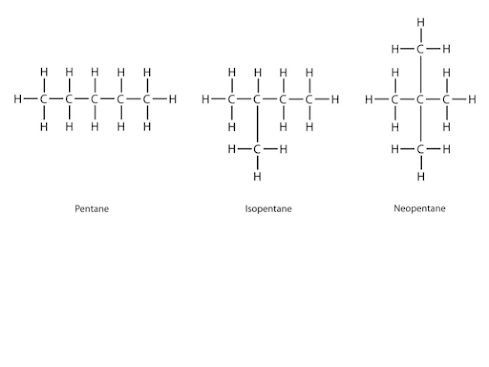12. A sample of vinegar has a pH of 5, and a sample of rainwater has a pH of 6. Which of the following statements best describes these two substances?
2 points
13. Which of the molecules below would be considered an alcohol?
2 points
diagram 5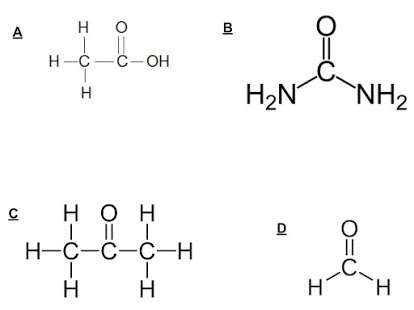14. What is the name of the compound with the formula CH3CH2CH3?
2 points
15. Alcohols contain what functional group?
2 points
16. Which of the following molecules could be built using ONLY single bonds and triple bonds?
2 points
17. Which of the molecules below is (are) considered an Alcohol?
2 points